• Call Now

1800-102-2727•

# Derivation of Equations of Motion - Algebraic, Calculus and Graphical Method

Motion, as we must understand, deals with moving objects.You get on your bike, you start pedaling slowly. You notice the speedometer attached to the front of the bike, it reads 10 km hr-1 After 10 s you notice that the reading changes to 20 km hr-1. The change in velocity of your bike indicates that you had accelerated in the process. You go to a car showroom, a flyer stuck on the car reads “ 0  to 40 km hr-1 in 4 s Such quick change in velocity must surely mean that the car has accelerated in the process. But how to find out this acceleration? Quantities like velocity, acceleration, displacement are needed to describe motion. The equations of motion help in determining their values. In this article, let us explore how to derive the 3 equations of motion through various methods.

## List of 3 Equations of Motion

The 3 equations of motion involving initial velocity u, displacement s, final velocity v, acceleration a and time t are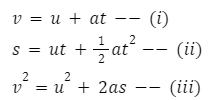Where v indicates the final velocity

u indicates the initial velocity

a indicates acceleration

t  indicates time and

s  indicates the displacement.

## Derivation of Equations of Motion Through Graphical Method

The first equation of motion can be derived using a graphical approach as follows.

Let’s take the following example: The velocity of a body changes from A to B in a time interval  t .A perpendicular is drawn from B  to C. BC = V indicates the final velocity.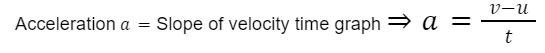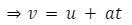Which gives us the first equation of motion.Area under the velocity time graph gives displacement.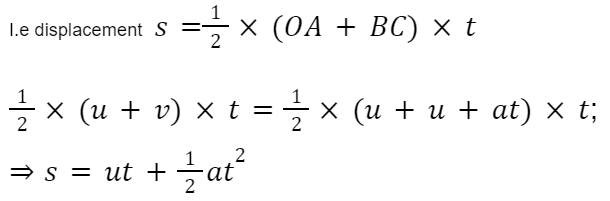Which gives us the second equation of motion.

From the graph, it is evident that distance or displacement covered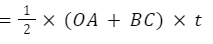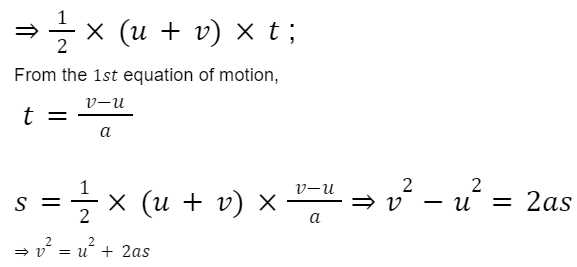Which gives us the third equation of motion.

## Derivation of Equations of Motion Through Calculus Method

In terms of differential equations, acceleration can be written as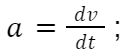Integrating both sides assigning limits (velocity changes from U to V and time changes from 0 to T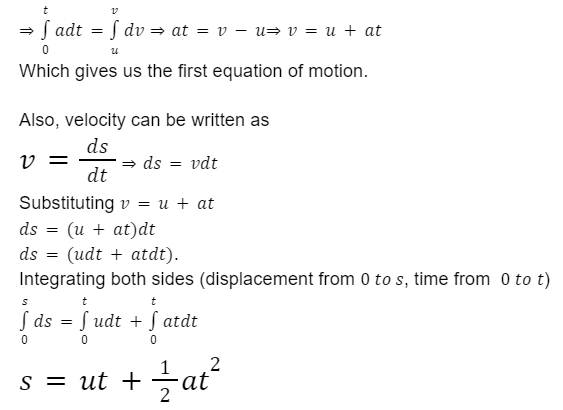Which gives us the second equation of motion.

The differential equation of acceleration a is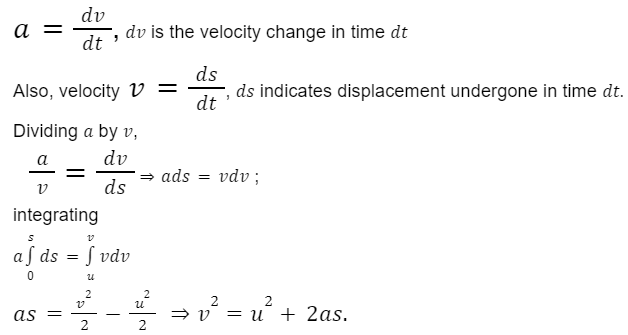Which gives us the third equation of motion.

## Derivation of Equations of Motion Through Algebraic Method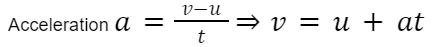Displacement s is the shortest possible path between two points. It is equal to the product of the average velocity and the time.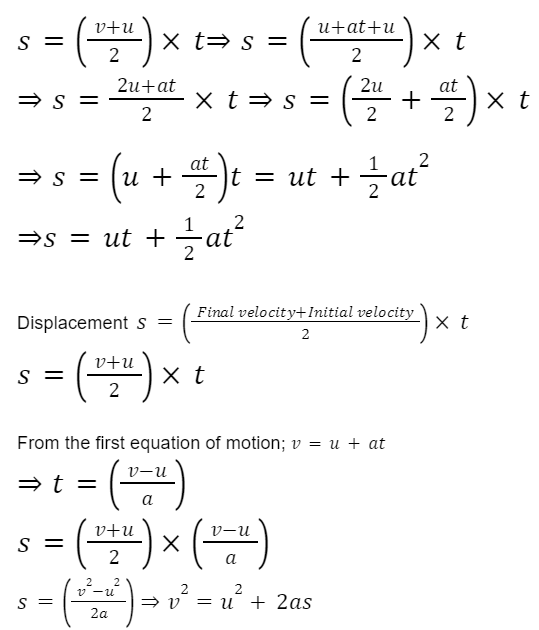Which gives us the third equation of motion.

## Practice Problems of Derivation of Equations of Motion

Question 1. A stone is dropped from height h and another from height 2h. The ratio of time taken by the stones to hit the ground is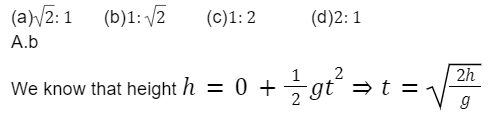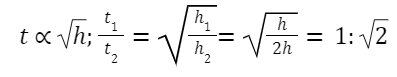Question 2. A ball falling from a Minaret covers 40 m in the last 2 seconds of its fall. Calculate height of the Minaret in meters?( g = 10 ms-2)

(a) 60           (b) 45             (c) 80       (d) 50

Let H be the height of the Minaret. Then distance traveled in (t - 2) seconds=(H - 40)m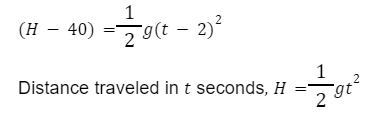Solving, we get 3s, H = 45m

Question 3. A bike traveling with a velocity of 80 Km/h slows down to 44 km/hr in 15 s. Find out the retardation?

(a) 0.67ms-1  (b) 1ms-1        (c)1.25ms-1     (d)1.5ms-1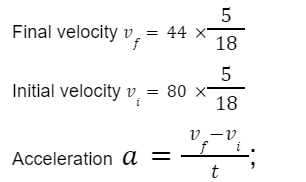Substituting and solving we getQuestion 4. A stone falls freely under gravity. It covers distances h1  hand h3 in the first 5 s, next 5 s and the next 5 s respectively,

Then h1  hand h3are related as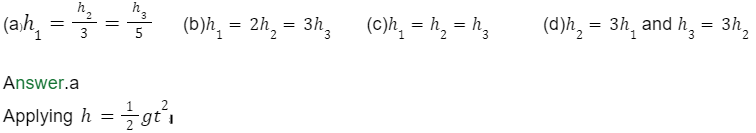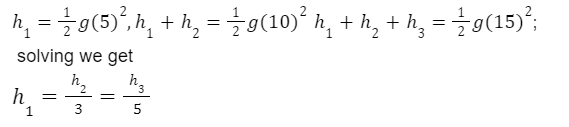Question 5. A stone is thrown upwards from a tower of height 60 m, with an initial speed of 20 ms-1.Taking upward direction as - ve, find the time taken by the stone to hit the ground. (g = 10ms-2)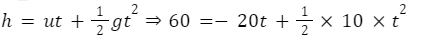Solving, we get t = 6s

Question 6. In the above example, find the time taken by the stone to reach the maximum height.

Answer: At the maximum height, the ball comes to rest.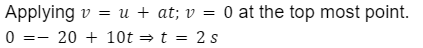Question 7. A stone is thrown vertically upwards. Its velocity after reaching half the height is 10 ms-1.Then, the maximum height reached by the stone is ( take g = 10ms-2)

(a)10 m           (b)16 m            (c)40 m            (d)20 m

Taking upward direction as positive.

If h is the maximum height reached by the stone; then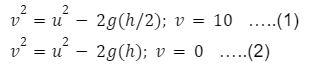Solving 1 and 2

∴ Total height= h = 10 m

Question 8. A stone is thrown upwards from the ground with an initial speed of 25.2 ms-1.How long does it take to reach its highest point? Also find height reached (g = 9.8 ms-2)

(a) 25.7 s, 34.2 m        (b)2.75 s, 3.24 m          (c)2.57 s, 32.4 m          27.5 s, 3.42 m

Taking upward direction as positive.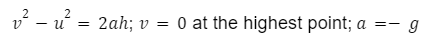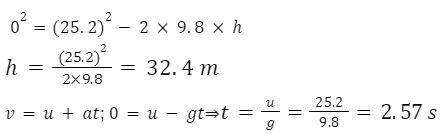## FAQs of Derivation of Equations of Motion

Question 1. A pebble is thrown upwards. What is the velocity when it reaches maximum height?

Answer:  We know that a pebble momentarily comes to rest at the highest point. So, velocity becomes zero.

Question 2.  What is the direction of acceleration due to gravity when a body is thrown downwards?

Answer: Acceleration due to gravity is always downwards no matter whether a body is thrown upwards or downwards.

Question 3. What is the third equation of motion?

Answer:  v2 = u2 + 2as

Where, v indicates the final velocity,

u indicates the initial velocity,

a indicates acceleration,

s  indicates displacement.

Question 4. When is displacement of a particle negative?

Answer: When it moves towards the negative direction of an axis, its displacement is - ve.

Question 5. What does the term a indicate in equations of motion?

Question 6. Can equations of motion be used in all situations?

Answer: No, equations of motion can only be used in case of constant acceleration.

## NCERT Class 11 Physics Chapters

 Physical World Units and Measurements Motion in a Straight Line Motion in a Plane Laws of Motion Work Energy and Power Particles and Rotational Motion Gravitation Mechanical Properties of Solids Mechanical Properties in Liquids Thermal Properties of Matter Thermodynamics Kinetic Theory Oscillations WavesTalk to our expert
Resend OTP Timer =
By submitting up, I agree to receive all the Whatsapp communication on my registered number and Aakash terms and conditions and privacy policy